# Tricky Data Sufficiency Questions: Explanations #1 and #2

Today, we present the explanations for the first two questions in our Tricky Data Sufficiency Questions post. Let’s get started.

## Question #1

1. What is the value of x?

(1) 5

x

+ 3

y

= 15

(2)

y

= 5 – (5/3)

x

(A) Statement (1) ALONE is sufficient, but statement (2) alone is not sufficient.

(B) Statement (2) ALONE is sufficient, but statement (1) alone is not sufficient.

(C) BOTH statements TOGETHER are sufficient, but NEITHER statement ALONE is sufficient.

(D) EACH statement ALONE is sufficient.

(E) Statements (1) and (2) TOGETHER are NOT sufficient

(hint)

Since (1) is a linear equation with two variables, it will allow you to solve for x only in terms of y. So that statement is insufficient by itself, eliminate answers A and D. This leaves B, C, and E.

Since (2) is also a linear equation with two variables, it will allow you to solve for x only in terms of y. So that statement is insufficient by itself, eliminate B. This leaves C and E.

You might be tempted to choose C, because (1) and (2) together give you two linear equations with the same two variables. As we’ve seen though, we must first make sure that the two equations are distinct. To see whether they are in fact the same equation in different guises, rewrite one of them—say Statement (1)—in the form that other already has.

So let’s solve for y using (1).

5x + 3y = 15

Subtract 5x from each side.

3y=15-5x

Divide each side by 3.

y = 5 – (5/3)x

So (1) and (2) are in fact the same equation. The correct answer is E.

Rather than checking in this way to see whether the two equations are equivalent, you could instead solve the system of equation by substitution, especially since (2) has already isolated y.

Take the value of y given in Statement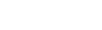and substitute that value for y in Statement (1).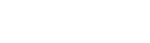Distribute the 3 to clear the parentheses.

5x – 15 + 5x = 15

Subtract 15 to each side to isolate the x terms on the left-hand side of the equation.

5x – 5x = 15 – 15

Simplify.

0=0

That doesn’t give us any information, so the correct answer is E.

## Question #2

2. If y = 2(x – 1) what is the value of x + y?

(1)

x

+ (6/

y

) = 3

(2)

y

=

x

+ 2

(A) Statement (1) ALONE is sufficient, but statement (2) alone is not sufficient.

(B) Statement (2) ALONE is sufficient, but statement (1) alone is not sufficient.

(C) BOTH statements TOGETHER are sufficient, but NEITHER statement ALONE is sufficient.

(D) EACH statement ALONE is sufficient.

(E) Statements (1) and (2) TOGETHER are NOT sufficient

(hint)

There’s no obviously useful way to rephrase the question, so we’ll probably need to solve for x and y and then add the values together. Keep an eye open, though, for an opportunity to solve for the sum x + y directly.

You may want to simplify the given equation—the “if” equation—before turning to the statements. Distribute the 2 on the right-hand of the equation to clear the fraction: y = 2x – 2. Now turn to the statements.

You might notice right away that (1) has a variable in a denominator as well as a one outside any denominator. This means that it is not a linear equation and so that information that at first appears sufficient will not be.

Whether you notice that or not, you’ll want to start with the simpler statement (2). Well, that a pleasant surprise! Statement (2) is a linear equation, as is the “if” equation. So unless they’re equivalent equations, (2) should be sufficient. Since both the “if” equation and (2) already isolate y, solving by substitution means setting equal the right hand sides of each equation.

x + 2 = 2x – 2

Subtract x from and add 2 to each side.

x = 4

You could solve for y and add that to 4 to solve x + y, but there’s no need to. If you can see that substituting 4 for x in either of the original equations will yield some constant value for y, then you can conclude that (2) allows you to solve for x + y, and so that (2) is sufficient. Eliminate A, C, and E.

Now let’s turn to Statement (1). A system of a single linear equation (the “if” equation) and a single nonlinear equation (Statement (1)) will not usually yield a unique constant value for each variable, but if we have the time to attempt a solution we should, unless we can rule out the exceptional cases some other way.

Since the “if” equation gives us y in terms of x, we can substitute 2x -2 for y in Statement (1).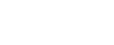Simplify the fraction by dividing the numerator and denominator by 2.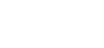Multiply all terms by to clear the fraction.

x (x – 1) + 3 = 3 (x – 1)

Distribute to clear the parentheses.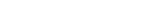Transpose to arrange in the usual quadratic form.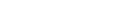Because this is a quadratic but not a quadratic square, it will have two solutions. Eliminate D. The correct answer is B.

Remember to check back on Monday for the explanations to questions #3 and #4. In the meantime, you can check your answers here. Have a good weekend!

## Author

•Michael Schwartz is really good at standardized tests. He’s earned multiple perfect scores on the GRE, GMAT, and LSAT. He’d rather have perfect pitch or be able to run low 1:40s for the 800 meters, but you take what you get. He has decades of teaching and curriculum-development experience. One of these days he might finish his dissertation and collect that Ph.D. in philosophy. Might.